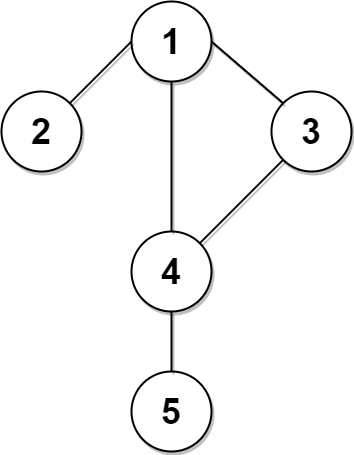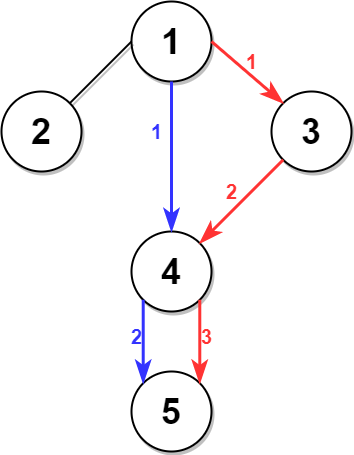# Leetcode 2045 - Second Minimum Time to Reach Destination

Note:

• Find the shortest path/second shortest path always involves BFS.
• Apprently, we need to record every vertex’s min and second min path from vertex 1.
• Construct a graph using an array and add every vertex with its neighbour.
• Create another array recording min and second min dist from 1 to it. Initialized as INF.
• Then write a classic BFS template.
• Note that when we compare curr dist with min and second min, if curr dist is bigger than second min, there is no need to add curr vertex to the queue again because it’s sure longer than the second longest path. If we don’t do that, we will end up with a loop as well.
• Finally, now we have path[n] which is the second longest path from 1 to n.
• Use a little bit math here, we use a for loop from 0 to secondMin - 1 to simulate the timelapse, if t % 2*change < change, it means we haven’t been into the wating time for the signal. If t % 2*change >= change, then we have to wait 2 * change - t % 2 * change.
•Question:

A city is represented as a bi-directional connected graph with n vertices where each vertex is labeled from 1 to n (inclusive). The edges in the graph are represented as a 2D integer array edges, where each edges[i] = [ui, vi] denotes a bi-directional edge between vertex ui and vertex vi. Every vertex pair is connected by at most one edge, and no vertex has an edge to itself. The time taken to traverse any edge is time minutes.

Each vertex has a traffic signal which changes its color from green to red and vice versa every change minutes. All signals change at the same time. You can enter a vertex at any time, but can leave a vertex only when the signal is green. You cannot wait at a vertex if the signal is green.

The second minimum value is defined as the smallest value strictly larger than the minimum value.

• For example the second minimum value of [2, 3, 4] is 3, and the second minimum value of [2, 2, 4] is 4.

Given n, edges, time, and change, return the second minimum time it will take to go from vertex 1 to vertex n.

Notes:

• You can go through any vertex any number of times, including 1 and n.
• You can assume that when the journey starts, all signals have just turned green.

Example:Code: